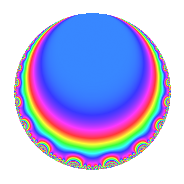# Properties

 Label 4012.2.a.eLevel 4012 Weight 2 Character orbit 4012.a Self dual Yes Analytic conductor 32.036 Analytic rank 1 Dimension 2 CM No Inner twists 1

# Related objects

## Newspace parameters

 Level: $$N$$ = $$4012 = 2^{2} \cdot 17 \cdot 59$$ Weight: $$k$$ = $$2$$ Character orbit: $$[\chi]$$ = 4012.a (trivial)

## Newform invariants

 Self dual: Yes Analytic conductor: $$32.0359812909$$ Analytic rank: $$1$$ Dimension: $$2$$ Coefficient field: $$\Q(\sqrt{5})$$ Coefficient ring: $$\Z[a_1, a_2, a_3]$$ Coefficient ring index: $$2$$ Fricke sign: $$1$$ Sato-Tate group: $\mathrm{SU}(2)$

## $q$-expansion

Coefficients of the $$q$$-expansion are expressed in terms of $$\beta = \sqrt{5}$$. We also show the integral $$q$$-expansion of the trace form.

 $$f(q)$$ $$=$$ $$q$$ $$-\beta q^{3}$$ $$- q^{5}$$ $$+ \beta q^{7}$$ $$+ 2 q^{9}$$ $$+O(q^{10})$$ $$q$$ $$-\beta q^{3}$$ $$- q^{5}$$ $$+ \beta q^{7}$$ $$+ 2 q^{9}$$ $$-2 \beta q^{11}$$ $$+ ( -1 + \beta ) q^{13}$$ $$+ \beta q^{15}$$ $$- q^{17}$$ $$+ 3 \beta q^{19}$$ $$-5 q^{21}$$ $$-2 \beta q^{23}$$ $$-4 q^{25}$$ $$+ \beta q^{27}$$ $$+ q^{29}$$ $$+ 6 q^{31}$$ $$+ 10 q^{33}$$ $$-\beta q^{35}$$ $$+ ( -5 + 3 \beta ) q^{37}$$ $$+ ( -5 + \beta ) q^{39}$$ $$+ ( -3 + 2 \beta ) q^{41}$$ $$+ ( -4 - 2 \beta ) q^{43}$$ $$-2 q^{45}$$ $$-2 q^{47}$$ $$-2 q^{49}$$ $$+ \beta q^{51}$$ $$+ ( 5 - 2 \beta ) q^{53}$$ $$+ 2 \beta q^{55}$$ $$-15 q^{57}$$ $$+ q^{59}$$ $$+ ( 7 + 3 \beta ) q^{61}$$ $$+ 2 \beta q^{63}$$ $$+ ( 1 - \beta ) q^{65}$$ $$+ ( -6 + 4 \beta ) q^{67}$$ $$+ 10 q^{69}$$ $$+ ( -2 - 2 \beta ) q^{71}$$ $$+ 6 \beta q^{73}$$ $$+ 4 \beta q^{75}$$ $$-10 q^{77}$$ $$+ ( -2 - 3 \beta ) q^{79}$$ $$-11 q^{81}$$ $$+ ( -1 - \beta ) q^{83}$$ $$+ q^{85}$$ $$-\beta q^{87}$$ $$+ ( 2 - 2 \beta ) q^{89}$$ $$+ ( 5 - \beta ) q^{91}$$ $$-6 \beta q^{93}$$ $$-3 \beta q^{95}$$ $$+ ( -1 + 3 \beta ) q^{97}$$ $$-4 \beta q^{99}$$ $$+O(q^{100})$$ $$\operatorname{Tr}(f)(q)$$ $$=$$ $$2q$$ $$\mathstrut -\mathstrut 2q^{5}$$ $$\mathstrut +\mathstrut 4q^{9}$$ $$\mathstrut +\mathstrut O(q^{10})$$ $$2q$$ $$\mathstrut -\mathstrut 2q^{5}$$ $$\mathstrut +\mathstrut 4q^{9}$$ $$\mathstrut -\mathstrut 2q^{13}$$ $$\mathstrut -\mathstrut 2q^{17}$$ $$\mathstrut -\mathstrut 10q^{21}$$ $$\mathstrut -\mathstrut 8q^{25}$$ $$\mathstrut +\mathstrut 2q^{29}$$ $$\mathstrut +\mathstrut 12q^{31}$$ $$\mathstrut +\mathstrut 20q^{33}$$ $$\mathstrut -\mathstrut 10q^{37}$$ $$\mathstrut -\mathstrut 10q^{39}$$ $$\mathstrut -\mathstrut 6q^{41}$$ $$\mathstrut -\mathstrut 8q^{43}$$ $$\mathstrut -\mathstrut 4q^{45}$$ $$\mathstrut -\mathstrut 4q^{47}$$ $$\mathstrut -\mathstrut 4q^{49}$$ $$\mathstrut +\mathstrut 10q^{53}$$ $$\mathstrut -\mathstrut 30q^{57}$$ $$\mathstrut +\mathstrut 2q^{59}$$ $$\mathstrut +\mathstrut 14q^{61}$$ $$\mathstrut +\mathstrut 2q^{65}$$ $$\mathstrut -\mathstrut 12q^{67}$$ $$\mathstrut +\mathstrut 20q^{69}$$ $$\mathstrut -\mathstrut 4q^{71}$$ $$\mathstrut -\mathstrut 20q^{77}$$ $$\mathstrut -\mathstrut 4q^{79}$$ $$\mathstrut -\mathstrut 22q^{81}$$ $$\mathstrut -\mathstrut 2q^{83}$$ $$\mathstrut +\mathstrut 2q^{85}$$ $$\mathstrut +\mathstrut 4q^{89}$$ $$\mathstrut +\mathstrut 10q^{91}$$ $$\mathstrut -\mathstrut 2q^{97}$$ $$\mathstrut +\mathstrut O(q^{100})$$

## Embeddings

For each embedding $$\iota_m$$ of the coefficient field, the values $$\iota_m(a_n)$$ are shown below.

For more information on an embedded modular form you can click on its label.

Label $$\iota_m(\nu)$$ $$a_{2}$$ $$a_{3}$$ $$a_{4}$$ $$a_{5}$$ $$a_{6}$$ $$a_{7}$$ $$a_{8}$$ $$a_{9}$$ $$a_{10}$$
1.1
 1.61803 −0.618034
0 −2.23607 0 −1.00000 0 2.23607 0 2.00000 0
1.2 0 2.23607 0 −1.00000 0 −2.23607 0 2.00000 0
 $$n$$: e.g. 2-40 or 990-1000 Significant digits: Format: Complex embeddings Normalized embeddings Satake parameters Satake angles

## Inner twists

This newform does not admit any (nontrivial) inner twists.

## Atkin-Lehner signs

$$p$$ Sign
$$2$$ $$-1$$
$$17$$ $$1$$
$$59$$ $$-1$$

## Hecke kernels

This newform can be constructed as the intersection of the kernels of the following linear operators acting on $$S_{2}^{\mathrm{new}}(\Gamma_0(4012))$$:

 $$T_{3}^{2}$$ $$\mathstrut -\mathstrut 5$$ $$T_{5}$$ $$\mathstrut +\mathstrut 1$$